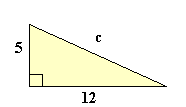# Pythagoras theorem

Geometry Level 1In mathematics, the Pythagorean theorem—or Pythagoras' theorem—is a relation in Euclidean geometry among the three sides of a right triangle.

Solve this triangle and comment your solution below.

×